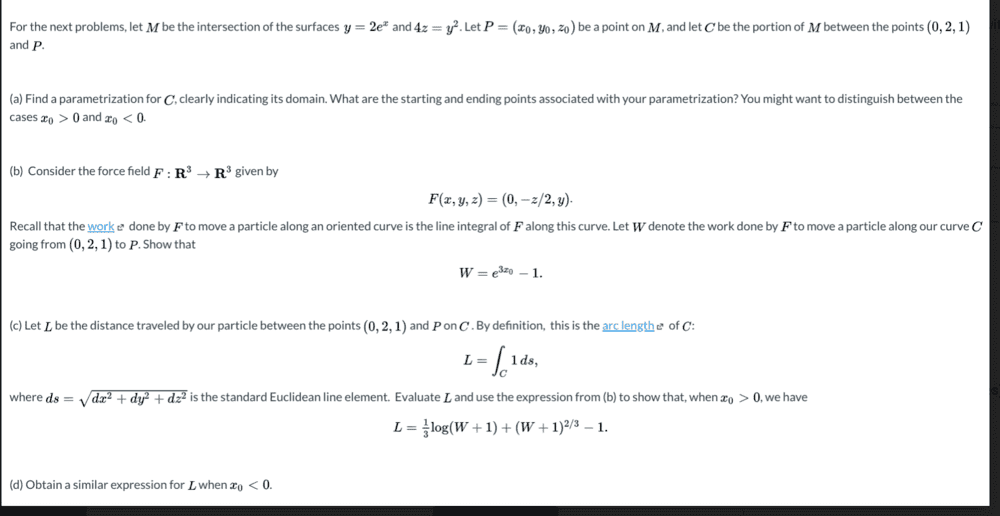# Multivariable Arc Length Problem: Weird Form with Parameterization

Homework Statement:
See Attachment
Relevant Equations:
ds=sqrt(dx^2+dy^2+dz^2)
Arc Length = integral on C of ds
Problem: See Attachment. Parts (a) & (b) are clear, but my confusion arises in (c)-- I feel like there is a much simpler form. While technically my answer is correct, there must be something I'm missing.

I parameterized the curve C=(t, e^2t, e^2t) and got c'(t)=(1,2e^2t,2e^2t), which should be correct since I got the answer for (b). I said ds=sqrt(||c'(t)||)

So ds=sqrt(1+4e^2t+4e^4t) =sqrt((1+2e^2t)^2)=1 + 2e^2t. The limits are from t=0 to t=x_0.

Integral(1+2e^2t) dt = t+e^2t, and I'll evaluate at the limits to get:

x_0 + e^(2x_0) - 1. So if x_0=ln(W+1)^(1/3), then e^(2x_0)= (W+1)^2/3. But still, why ask for it in this crazy form? Or is it just a silly excuse tobenorin
Homework Helper
Gold Member
So ds=sqrt(1+4e^2t+4e^4t) =sqrt((1+2e^2t)^2)=1 + 2e^2t.
Stop right there, check your formula, my clac book says that

$$ds = \sqrt{\left( \tfrac{dx}{dt}\right) ^2 +\left( \tfrac{dy}{dt}\right) ^2 +\left( \tfrac{dz}{dt}\right) ^2 } \, dt$$

•etotheipi
Stop right there, check your formula, my clac book says that

$$ds = \sqrt{\left( \tfrac{dx}{dt}\right) ^2 +\left( \tfrac{dy}{dt}\right) ^2 +\left( \tfrac{dz}{dt}\right) ^2 } \, dt$$

I agree on the formula, but not sure where I went wrong. Since it's arc length and the path is parameterized as c(t)= (t, 2e^t, e^(2t)) and c'(t)= (1, 2e^(t), 2e^(2t)) = (dx/dt, dy/dt, dz/dt). So squaring all terms and summing up gives: 1 + 4e^(2t) + 4e^(4t). So then I took the square root, simplified to 1+2e^2t, and then tacked on the dt. Where did I go wrong?

Mark44
Mentor
Stop right there, check your formula, my clac book says that

##ds = \sqrt{\left( \tfrac{dx}{dt}\right) ^2 +\left( \tfrac{dy}{dt}\right) ^2 +\left( \tfrac{dz}{dt}\right) ^2 } \, dt##
The above is equivalent to ##ds = \sqrt{dx^2 + dy^2 + dz^2}##